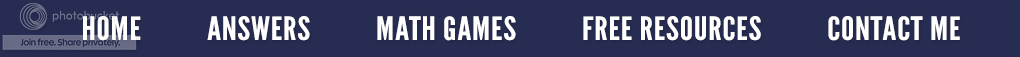Is zero even? What an "odd" question!

My daughter and her husband are heading to Las Vegas with his family to celebrate his parent's 50th wedding anniversary.  I guess when you are at the roulette table, (never been there or done that) and you bet "even" and the little ball lands on 0 or 00, you lose. Yep, it's true; zero is not considered an even number on the roulette wheel, something you better know before you bet.  This example is a non-mathematical, real-life situation where zero is neither odd or even.  But in mathematics, by definition, zero is an even number. (An even number is any number that can be exactly divided by 2 with no remainder.)  In other words, an odd number leaves a remainder of 1 when divided by 2 whereas an even number has nothing left over.  Under this definition, zero is definitely an even number since 0 ÷ 2 = 0  has no remainder.

Zero also fits the pattern when you count which is the same as alternating even (E) and odd (O) numbers.
Most math books include zero as an even number; however, under special circumstances zero may be excluded.  (For example, when defining even numbers to mean even NATURAL numbers.)  Natural numbers are the set of counting numbers beginning with 1 {1, 2, 3, 4, 5....}; so, zero is not included.
Consider the following simple illustrations.  Let's put some numbers in groups of two and see what happens.

As you can see, even numbers such as 4 have no "odd man out" whereas odd numbers such as 3 always have one left over.  Similarly, when zero is split into two groups, there is not a single star that does not fit into one of the two groups.  Each group contains no stars or exactly the same amount.  Consequently, zero is even.

Algebraically, we can write even numbers as 2n where n is an integer while odd numbers are written as 2n + 1 where "n" is an integer.  If n = 0, then 2n = 2 x 0 = 0 (even) and 2n + 1 = 2 x 0 + 1 = 1 (odd).  All integers are either even or odd. (This is a theorem).  Zero is not odd because it cannot fit the form 2n + 1 where "n" is an integer. Therefore, since it is not odd, it must be even.

I know this seems much ado about nothing, but a great deal of discussion has surrounded this very fact on the college level.  Some instructors feel zero is neither odd or even.  (Yes, we like to debate things that seem obvious to others.)

Consider this multiple choice question.  (It might just appear on some important standardized test.)Which answer would you choose and why?

Zero is…
a.) even
b.) odd
c.) all of the above
d.) none of the above

Mathematically, I see zero as the count of no objects, or in more formal terms, it is the number of objects in the empty set.  Also, since zero is defined as an even number in most math textbooks, and is divisible by 2 with no remainder, then "a" is my answer.